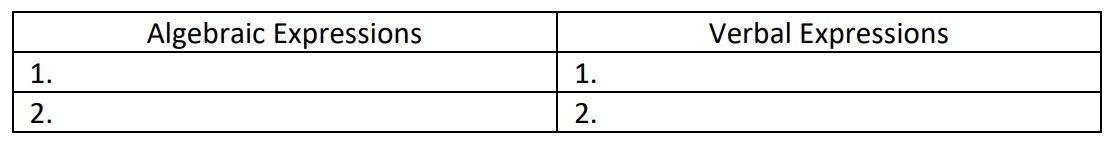# 5th Grade, Activity 16: Write and Interpret Numerical Expressions

Write simple expressions that record calculations with whole numbers, fractions, and decimals, and interpret numerical expressions without evaluating them.

For example, express the calculation “add and 7, then multiply by 2” as 2 × (8 + 7). Recognize that 3 x (18,932 + 9.21) is three times as large as 18,932 + 9.21, without having to calculate the indicated sum or product.

### Write and Interpret Expressions

• Group: Whole Class/Alone/Pair
• Materials: paper and pencil, Write and Interpret Expressions, half sheets A, B, C, & D

Explain that students will write verbal expressions for each algebraic expression and vice versa. Cut the halfsheets along the dotted lines. Distribute half sheet A to half of the class. Issue half sheet B to the other half of the class. The group with half sheet A must write verbal expressions for the algebraic expressions. The group with half sheet B must write algebraic expressions for the verbal expressions. Write two columns on the board as shown below. After the first round, invite two students at a time to write either the verbal expression or the algebraic expression on the board. For example, two students are invited to the board at the same time. One student writes the verbal expression for #1 and the other student writes the algebraic expression for #1. Both verbal and algebraic expression cards should correspond.Leave the columns on the board for the second distribution. Distribute half sheet C to the students that were issued half sheet A. Issue half sheet D to the students that were issued half sheet B. The group with half sheet C must write algebraic expressions for the verbal expressions. The group with half sheet D must write verbal expressions for the algebraic expressions. Continue the second round in the same manner as in the first round. After the second round, invite two students at a time to write the answers for the rest of the expressions in the chart.